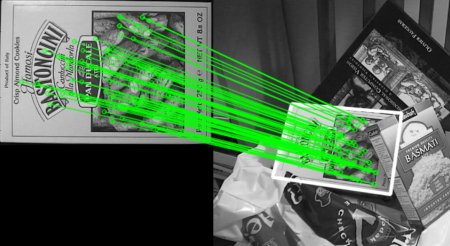# OpenCV-Python 系列 四十四 | 特征匹配 + 单应性查找对象

### 目标

– 我们将把calib3d模块中的特征匹配和findHomography混合在一起，以在复杂图像中找到已知对象。

### 代码

import numpy as np
import cv2 as cv
from matplotlib import pyplot as plt
MIN_MATCH_COUNT = 10
# 初始化SIFT检测器
sift = cv.xfeatures2d.SIFT_create()
# 用SIFT找到关键点和描述符
kp1, des1 = sift.detectAndCompute(img1,None)
kp2, des2 = sift.detectAndCompute(img2,None)
FLANN_INDEX_KDTREE = 1
index_params = dict(algorithm = FLANN_INDEX_KDTREE, trees = 5)
search_params = dict(checks = 50)
flann = cv.FlannBasedMatcher(index_params, search_params)
matches = flann.knnMatch(des1,des2,k=2)
# ＃根据Lowe的比率测试存储所有符合条件的匹配项。
good = []
for m,n in matches:
if m.distance < 0.7*n.distance:
good.append(m)


if len(good)>MIN_MATCH_COUNT:
src_pts = np.float32([ kp1[m.queryIdx].pt for m in good ]).reshape(-1,1,2)
dst_pts = np.float32([ kp2[m.trainIdx].pt for m in good ]).reshape(-1,1,2)
M, mask = cv.findHomography(src_pts, dst_pts, cv.RANSAC,5.0)
h,w,d = img1.shape
pts = np.float32([ [0,0],[0,h-1],[w-1,h-1],[w-1,0] ]).reshape(-1,1,2)
dst = cv.perspectiveTransform(pts,M)
img2 = cv.polylines(img2,[np.int32(dst)],True,255,3, cv.LINE_AA)
else:
print( "Not enough matches are found - {}/{}".format(len(good), MIN_MATCH_COUNT) )


draw_params = dict(matchColor = (0,255,0), # 用绿色绘制匹配
singlePointColor = None,
flags = 2)
img3 = cv.drawMatches(img1,kp1,img2,kp2,good,None,**draw_params)
plt.imshow(img3, 'gray'),plt.show()